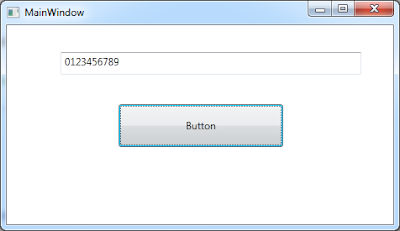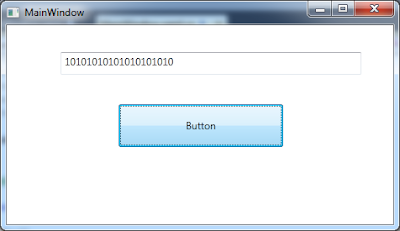## Tuesday, December 7, 2010

### WPF - Dispatcher.BeginInvoke and Closures‏

In one of my previous posts I discussed about the issues with using Dispatcher.Invoke. In this post, we are discussing some possible issues with Dispatcher.BeginInvoke and Closures. Let us start with an example and see the expected result. Then we will be discussing how we can improve the results.
`<Window x:Class="CapturedVariableLambda_BeginInvoke.MainWindow"        xmlns="http://schemas.microsoft.com/winfx/2006/xaml/presentation"        xmlns:x="http://schemas.microsoft.com/winfx/2006/xaml"        Title="MainWindow" Height="275" Width="476">    <Grid>        <TextBox Height="27" HorizontalAlignment="Left" Margin="64,32,0,0"                 Name="myTextBox" VerticalAlignment="Top" Width="358" />        <Button Content="Button" Height="51" HorizontalAlignment="Left"                Margin="133,94,0,0" Name="myButton" VerticalAlignment="Top"Width="196" Click="myButton_Click" />    </Grid></Window>`

Let us update the code behind with the definition of myButton_Click handler.
`public partial class MainWindow : Window{    public MainWindow()    {        InitializeComponent();    }     private void myButton_Click(object sender, RoutedEventArgs e)    {        Thread t = new Thread(() =>                                  {                                      for (int i = 0; i <= 9; i++)                                      {                                          myTextBox.Dispatcher.BeginInvoke(                                              new Action(() => myTextBox.Text =                                                  string.Format("{0}{1}", myTextBox.Text, i.ToString())));                                      }                                  });         t.IsBackground = true;        t.Start();    }}`

You probably are imagining the textbox to be updated as follows when we click the button.But as we click the button, the text box is updated as follow:Hmm! It's interesting, but why? Wanna know? Let us discuss!

Basically this is a known behavior of Captured variables. They are outer variables used by lambda expression / statement. These types of lambda expressions / statements are called Closures. As we all know there is delayed execution of lambda expression. While being executed, they use the current value of the captured variable rather than the value when they were created.

In order to solve this problem of closure, there is a universal remedy. Whenever we need to capture a variable in a closure, we must be making a local copy of the variable by declaring a local variable in lambda and assigning the value of captured variable to this local variable.

Let us update our code based on above explanation and see if that results in expected result. Let us update the handler for button click event as follows:
`private void myButton_Click(object sender, RoutedEventArgs e){    Thread t = new Thread(() =>                              {                                  for (int i = 0; i <= 9; i++)                                  {                                      int iLocal = i;                                      myTextBox.Dispatcher.BeginInvoke(                                          new Action(() => myTextBox.Text =                                              string.Format("{0}{1}", myTextBox.Text, iLocal.ToString())));                                  }                              });     t.IsBackground = true;    t.Start();}`

Now we run the application and click the button. The form updates as follows.As you can see this is fixed now as per expectation.

Finally a word of advice: Always assign a captured variable to a local variable in Closure.